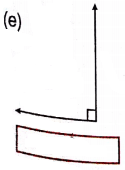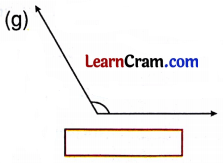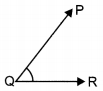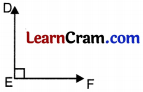# DAV Class 4 Maths Chapter 10 Worksheet 4 Solutions

The DAV Maths Book Class 4 Solutions and DAV Class 4 Maths Chapter 10 Worksheet 4 Solutions of Angles offer comprehensive answers to textbook questions.

## DAV Class 4 Maths Ch 10 WS 4 Solutions

Question 1.
Measure the following angles and verify them as acute, obtuse, right or straight angle.Acute AngleRight angleObtuse AngleAcute AngleRight AngleObtuse AngleObtuse AngleStraight AngleQuestion 2.
Draw the angles as per their types.
(a) ∠XYZ (Right angle)
∠XYZ(b) ∠PQR (Acute angle)
∠PQR(c) ∠MNP (Straight angle)